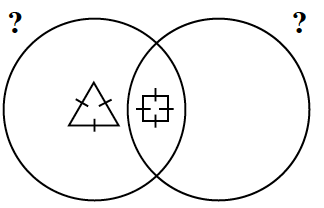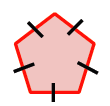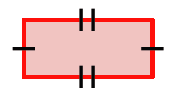Home > CCG > Chapter 2 > Lesson 2.1.1 > Problem2-12

2-12.

Larry saw Javon’s incomplete Venn diagram at right, and he wants to finish it. However, he does not know the condition that each circle represents. Find a possible label for each circle, and place two more shapes into the diagram. Homework Help ✎What do the two figures have in common? That trait is the condition the left circle represents.

What is a difference between the two shapes? The trait unique to the figure in the middle of the Venn diagram is the condition the right circle represents.

The left circle represents equilateral and the right circle represents quadrilateral.